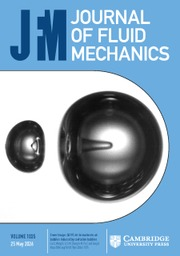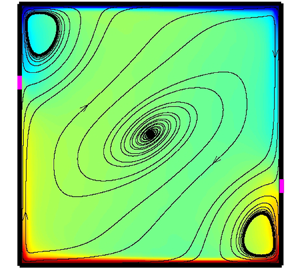Home
Hostname: page-component-78bd46657c-2pqp7 Total loading time: 1.179 Render date: 2021-05-10T08:05:17.242Z Has data issue: true Feature Flags: { "shouldUseShareProductTool": true, "shouldUseHypothesis": true, "isUnsiloEnabled": true, "metricsAbstractViews": false, "figures": false, "newCiteModal": false, "newCitedByModal": true }Journal of Fluid Mechanics

## AbstractIn this paper, we designed two different configurations with locally isothermal sidewalls, where the temperature is set to be the bulk temperature, to control the large-scale circulation in turbulent Rayleigh–Bénard convection, namely two-point control and four-point control. At fixed Rayleigh number$Ra=10^8$and Prandtl number$Pr=2$, a series of direct numerical simulations are performed on both two-dimensional (2-D) and quasi-two-dimensional (quasi-2-D) cavities with both types of control, where the width of the control area is fixed at$\delta _c=0.05$and the vertical distance from the cavity centre$h_c$ varies from 0 to 0.45 with an interval of 0.05. Our results show that the control effect depends on$h_c$, the control configurations as well as the flow dimensions. For 2-D cavities, both two-point control and four-point control suppress the flow reversal when$h_c \geq 0.05$, accompanied by the enhancement of vertical heat transfer and the strength of the large-scale circulation. For quasi-2-D cavities, the suppression of the flow reversals is obvious with two-point control and$h_c\geq 0.05$, while the effect is rather limited with four-point control. Further experiments with$Pr=5.7$ and$Ra$up to$7.36\times10^8$ show that two-point control with$h_c=0.15$can effectively suppress the flow reversal, while two-point control with$h_c=0$ can suppress the reversals at low$Ra=1.93\times 10^8$and activate them at higher$Ra=7.36\times 10^8$, which agrees well with our numerical simulations.

## JFM classification

Type
JFM Papers
Information
Journal of Fluid Mechanics , 25 May 2021 , A14

## Access options

Get access to the full version of this content by using one of the access options below.

## References

Ahlers, G., Grossmann, S. & Lohse, D. 2009 Heat transfer and large scale dynamics in turbulent Rayleigh–Bénard convection. Rev. Mod. Phys. 81 (2), 503537.CrossRefGoogle Scholar
Araujo, F.F., Grossmann, S. & Lohse, D. 2005 Wind reversals in turbulent Rayleigh–Bénard convection. Phys. Rev. Lett. 95, 084502.CrossRefGoogle ScholarPubMed
Assaf, M., Angheluta, L. & Goldenfeld, N. 2011 Rare fluctuations and large-scale circulation cessations in turbulent convection. Phys. Rev. Lett. 107, 044502.CrossRefGoogle ScholarPubMed
Benzi, R. 2005 Flow reversal in a simple dynamical model of turbulence. Phys. Rev. Lett. 95, 024502.CrossRefGoogle Scholar
Brown, E. & Ahlers, G. 2007 Large-scale circulation model for turbulent Rayleigh–Bénard convection. Phys. Rev. Lett. 98, 134501.CrossRefGoogle ScholarPubMed
Brown, E. & Ahlers, G. 2008 a Azimuthal asymmetries of the large-scale circulation in turbulent Rayleigh–Bénard convection. Phys. Fluids 20 (10), 105105.CrossRefGoogle Scholar
Brown, E. & Ahlers, G. 2008 b A model of diffusion in a potential well for the dynamics of the large-scale circulation in turbulent Rayleigh—Bénard convection. Phys. Fluids 20, 075101.CrossRefGoogle Scholar
Brown, E., Funfschilling, D. & Ahlers, G. 2007 Anomalous Reynolds-number scaling in turbulent Rayleigh–Bénard convection. J. Stat. Mech.-Theory Exp. 2007 (10), P10005.CrossRefGoogle Scholar
Brown, E., Nikolaenko, A. & Ahlers, G. 2005 Reorientation of the large-scale circulation in turbulent Rayleigh–Bénard convection. Phys. Rev. Lett. 95, 084503.CrossRefGoogle ScholarPubMed
Castillo-Castellanos, A., Sergent, A., Podvin, B. & Rossi, M. 2019 Cessation and reversals of large-scale structures in square Rayleigh–Bénard cells. J. Fluid Mech. 877, 922954.CrossRefGoogle Scholar
Chandra, M. & Verma, M.K. 2011 Dynamics and symmetries of flow reversals in turbulent convection. Phys. Rev. E 83, 067303.CrossRefGoogle ScholarPubMed
Chandra, M. & Verma, M.K. 2013 Flow reversals in turbulent convection via vortex reconnections. Phys. Rev. Lett. 110, 114503.CrossRefGoogle ScholarPubMed
Chen, X., Huang, S.-D., Xia, K.-Q. & Xi, H.-D. 2019 Emergence of substructures inside the large-scale circulation induces transition in flow reversals in turbulent thermal convection. J. Fluid Mech. 877, R1.CrossRefGoogle Scholar
Chen, X., Wang, D.-P. & Xi, H.-D. 2020 Reduced flow reversals in turbulent convection in the absence of corner vortices. J. Fluid Mech. 891, R5.CrossRefGoogle Scholar
Chong, K.L., Wagner, S., Kaczorowski, M., Shishkina, O. & Xia, K.-Q. 2018 Effect of Prandtl number on heat transport enhancement in Rayleigh–Bénard convection under geometrical confinement. Phys. Rev. Fluids 3, 013501.CrossRefGoogle Scholar
Cioni, S., Ciliberto, S. & Sommeria, J. 1997 Strongly turbulent Rayleigh—Bénard convection in mercury: comparison with results at moderate Prandtl number. J. Fluid Mech. 335, 111140.CrossRefGoogle Scholar
van Doorn, E., Dhruva, B., Sreenivasan, K.R. & Cassella, V. 2000 Statistics of wind direction and its increments. Phys. Fluids 12 (6), 15291534.CrossRefGoogle Scholar
Glatzmaiers, G.A. & Roberts, P.H. 1995 A three-dimensional self-consistent computer simulation of a geomagnetic field reversal. Nature 377 (6546), 203209.CrossRefGoogle Scholar
Grossmann, S. & Lohse, D. 2000 Scaling in thermal convection: a unifying theory. J. Fluid Mech. 407, 2756.CrossRefGoogle Scholar
Grossmann, S. & Lohse, D. 2001 Thermal convection for large Prandtl numbers. Phys. Rev. Lett. 86, 33163319.CrossRefGoogle ScholarPubMed
Grossmann, S. & Lohse, D. 2002 Prandtl and Rayleigh number dependence of the Reynolds number in turbulent thermal convection. Phys. Rev. E 66, 016305.CrossRefGoogle ScholarPubMed
Hartmann, D.L., Moy, L.A. & Fu, Q. 2001 Tropical convection and the energy balance at the top of the atmosphere. J. Climate 14 (24), 44954511.2.0.CO;2>CrossRefGoogle Scholar
Huang, S.-D., Wang, F., Xi, H.-D. & Xia, K.-Q. 2015 Comparative experimental study of fixed temperature and fixed heat flux boundary conditions in turbulent thermal convection. Phys. Rev. Lett. 115, 154502.CrossRefGoogle ScholarPubMed
Huang, S.-D. & Xia, K.-Q. 2016 Effects of geometric confinement in quasi-2-d turbulent Rayleigh–Bénard convection. J. Fluid Mech. 794, 639654.CrossRefGoogle Scholar
Kooij, G., Botchev, M., Frederix, E., Geurts, B., Horn, S., Lohse, D., van der Poel, E., Shishkina, O., Stevens, R.J. & Verzicco, R. 2018 Comparison of computational codes for direct numerical simulations of turbulent Rayleigh–Bénard convection. Comput. Fluids 166, 18.CrossRefGoogle Scholar
Lohse, D. & Xia, K.-Q. 2010 Small-scale properties of turbulent Rayleigh–Bénard convection. Annu. Rev. Fluid Mech. 42, 335364.CrossRefGoogle Scholar
Ni, R., Huang, S.-D. & Xia, K.-Q. 2015 Reversals of the large-scale circulation in quasi-2D Rayleigh–Bénard convection. J. Fluid Mech. 778, R5.CrossRefGoogle Scholar
Petschel, K., Wilczek, M., Breuer, M., Friedrich, R. & Hansen, U. 2011 Statistical analysis of global wind dynamics in vigorous Rayleigh–Bénard convection. Phys. Rev. E 84, 026309.CrossRefGoogle ScholarPubMed
Podvin, B. & Sergent, A. 2015 A large-scale investigation of wind reversal in a square Rayleigh–Bénard cell. J. Fluid Mech. 766, 172201.CrossRefGoogle Scholar
Qiu, X.-L., Xia, K.-Q. & Tong, P. 2005 Experimental study of velocity boundary layer near a rough conducting surface in turbulent natural convection. J. Turbul. 6, N30.CrossRefGoogle Scholar
Sreenivasan, K., Bershadskii, A. & Niemela, J.J. 2002 Mean wind and its reversal in thermal convection. Phys. Rev. E 65, 056306.CrossRefGoogle ScholarPubMed
Stevens, R.J., Lohse, D. & Verzicco, R. 2014 Sidewall effects in Rayleigh–Bénard convection. J. Fluid Mech. 741, 127.CrossRefGoogle Scholar
Sugiyama, K., Ni, R., Stevens, R.J., Chan, T.S., Zhou, S.-Q., Xi, H.-D., Sun, C., Grossmann, S., Xia, K.-Q. & Lohse, D. 2010 Flow reversals in thermally driven turbulence. Phys. Rev. Lett. 105, 034503.CrossRefGoogle ScholarPubMed
Sun, C., Xi, H.-D. & Xia, K.-Q. 2005 Azimuthal symmetry, flow dynamics, and heat transport in turbulent thermal convection in a cylinder with an aspect ratio of 0.5. Phys. Rev. Lett. 95, 074502.CrossRefGoogle Scholar
Tsuji, Y., Mizuno, T., Mashiko, T. & Sano, M. 2005 Mean wind in convective turbulence of mercury. Phys. Rev. Lett. 94, 034501.CrossRefGoogle ScholarPubMed
Van Der Poel, E.P., Ostilla-Mónico, R., Donners, J. & Verzicco, R. 2015 A pencil distributed finite difference code for strongly turbulent wall-bounded flows. Comput. Fluids 116, 1016.CrossRefGoogle Scholar
Vasil'ev, A. & Frick, P.G. 2011 Reversals of large-scale circulation in turbulent convection in rectangular cavities. J. Expl Theor. Phys. 93, 330334.CrossRefGoogle Scholar
Wagner, S. & Shishkina, O. 2013 Aspect-ratio dependency of Rayleigh–Bénard convection in box-shaped containers. Phys. Fluids 25, 085110.CrossRefGoogle Scholar
Wan, Z.H., Wei, P., Verzicco, R., Lohse, D., Ahlers, G. & Stevens, R.J. 2019 Effect of sidewall on heat transfer and flow structure in Rayleigh–Bénard convection. J. Fluid Mech. 881, 218243.CrossRefGoogle Scholar
Wang, Q., Xia, S.-N., Wang, B.-F., Sun, D.-J., Zhou, Q. & Wan, Z.-H. 2018 Flow reversals in two-dimensional thermal convection in tilted cells. J. Fluid Mech. 849, 355372.CrossRefGoogle Scholar
Wang, Q., Xu, B.-L., Xia, S.-N., Wan, Z.-H. & Sun, D.-J. 2017 Thermal convection in a tilted rectangular cell with aspect ratio 0.5. Chin. Phys. Lett. 34, 104401.CrossRefGoogle Scholar
Wang, B.-F., Zhou, Q. & Sun, C. 2020 Vibration-induced boundary-layer destabilization achieves massive heat-transport enhancement. Sci. Adv. 6, eaaz8239.CrossRefGoogle ScholarPubMed
Xi, H.-D. & Xia, K.-Q. 2007 Cessations and reversals of the large-scale circulation in turbulent thermal convection. Phys. Rev. E 75, 066307.CrossRefGoogle ScholarPubMed
Xi, H.-D. & Xia, K.-Q. 2008 Azimuthal motion, reorientation, cessation, and reversal of the large-scale circulation in turbulent thermal convection: a comparative study in aspect ratio one and one-half geometries. Phys. Rev. E 78, 036326.CrossRefGoogle ScholarPubMed
Xia, S.-N., Wan, Z.-H., Liu, S., Wang, Q. & Sun, D.-J. 2016 Flow reversals in Rayleigh–Bénard convection with non-Oberbeck–Boussinesq effects. J. Fluid Mech. 798, 628642.CrossRefGoogle Scholar
Zhang, Y.-Z., Sun, C., Bao, Y. & Zhou, Q. 2018 How surface roughness reduces heat transport for small roughness heights in turbulent Rayleigh–Bénard convection. J. Fluid Mech. 836, R2.CrossRefGoogle Scholar
Zhang, S., Xia, Z., Zhou, Q. & Chen, S. 2020 Controlling flow reversal in two-dimensional Rayleigh–Bénard convection. J. Fluid Mech. 891, R4.CrossRefGoogle Scholar
Zhu, X., Stevens, R.J.A.M., Shishkina, O., Verzicco, R. & Lohse, D. 2019$Nu\sim Ra^{1/2}$ scaling enabled by multiscale wall roughness in Rayleigh–Bénard turbulence. J. Fluid Mech. 869, R4.CrossRefGoogle Scholar

# Send article to Kindle

Note you can select to send to either the @free.kindle.com or @kindle.com variations. ‘@free.kindle.com’ emails are free but can only be sent to your device when it is connected to wi-fi. ‘@kindle.com’ emails can be delivered even when you are not connected to wi-fi, but note that service fees apply.

Find out more about the Kindle Personal Document Service.

Stabilizing/destabilizing the large-scale circulation in turbulent Rayleigh–Bénard convection with sidewall temperature control
Available formats
×

# Send article to Dropbox

To send this article to your Dropbox account, please select one or more formats and confirm that you agree to abide by our usage policies. If this is the first time you use this feature, you will be asked to authorise Cambridge Core to connect with your <service> account. Find out more about sending content to Dropbox.

Stabilizing/destabilizing the large-scale circulation in turbulent Rayleigh–Bénard convection with sidewall temperature control
Available formats
×

# Send article to Google Drive

To send this article to your Google Drive account, please select one or more formats and confirm that you agree to abide by our usage policies. If this is the first time you use this feature, you will be asked to authorise Cambridge Core to connect with your <service> account. Find out more about sending content to Google Drive.

Stabilizing/destabilizing the large-scale circulation in turbulent Rayleigh–Bénard convection with sidewall temperature control
Available formats
×
×